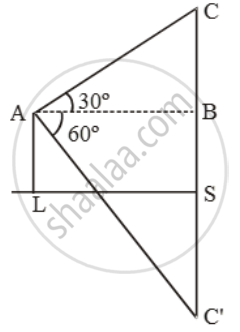# If the angle of elevation of cloud from a point 200 m above a lake is 30º and the angle of depression of its reflection in the lake is 60º, then find the height of the cloud above the lake - Mathematics

Sum

If the angle of elevation of cloud from a point 200 m above a lake is 30º and the angle of depression of its reflection in the lake is 60º, then find the height of the cloud above the lake

#### Solution

Let C be the cloud and C’ be its reflection in the lake.

Let CS = C’S = x.\text{Now, }\frac{BC}{AB}=\text{tan }30^\text{o}=\frac{1}{\sqrt{3}}

\Rightarrow x-200=\frac{AB}{\sqrt{3}}

\text{Also, }\frac{BC'}{AB}=\text{tan }60^\text{o}=\sqrt{3}

\Rightarrow x+200=(AB)\sqrt{3}.

\therefore \sqrt{3}(x-200)=\frac{x+200}{\sqrt{3}}\text{ or }x=\text{400}.

∴ CS = 400 m.

Concept: Heights and Distances
Is there an error in this question or solution?